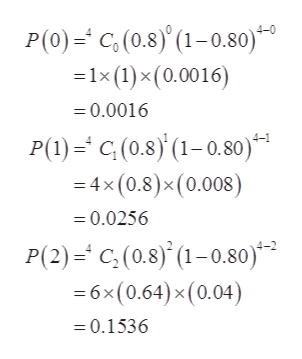# A certain newspaper reports that about 20% of all prison parolees become repeat offenders. Alice is a social worker whose job is to counsel people on parole. Let us say success means a person does not become a repeat offender. Alice has been given a group of four parolees.(a) Find the probability P(r) of r successes ranging from 0 to 4. (Round your answers to three decimal places.)P(0) =P(1) =P(2) =P(3) =P(4) =

Question
55 views

A certain newspaper reports that about 20% of all prison parolees become repeat offenders. Alice is a social worker whose job is to counsel people on parole. Let us say success means a person does not become a repeat offender. Alice has been given a group of four parolees.

(a) Find the probability P(r) of r successes ranging from 0 to 4. (Round your answers to three decimal places.)
 P(0) = P(1) = P(2) = P(3) = P(4) =

check_circle

Step 1

From the provided information, the probability that all prison parolees become repeat offenders is 0.20. So, the probability that all prison parolees do not become repeat offenders will be (1-0.20) 0.80, which is assumed as probability of success (p). Consider X be random variable which represents the number of parolees who does not become a repeat offender. The number of trials (n) is 4. The binomial distribution will be used for calculating the probability as:

Step 2

Now, the required probabilities for r=0...help_outlineImage TranscriptioncloseP(0)C,(0.8)'(1-0.80) -1x (1)x (0.0016) = 0.0016 P(1)C(0.8)(1-0.80) =4x(0.8)x (0.008) =0.0256 P(2)=C,(0.8) (1-0.80) 6x (0.64) x(0.04) =0.1536 fullscreen

### Want to see the full answer?

See Solution

#### Want to see this answer and more?

Solutions are written by subject experts who are available 24/7. Questions are typically answered within 1 hour.*

See Solution
*Response times may vary by subject and question.
Tagged in

### Probability